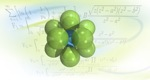0

## Mathematical Humor

Published on Thursday, March 01, 2007 in , , ,Sure, Mathworld is a great resource for learning about things like Pi, but that doesn't mean it's all dry and boring. As a matter of fact, if you look closely, Mathworld is a surprisingly amusing site.

It was the Athanasuis Kircher Society that first alerted me to Tupper's Self-Referential Formula. This unusual formula, when plotted on a graphing calculator will form an image of the formula itself!

Next, Mikhail Klassen alerted me to three different amusing numerical laws:

Strong Law of Small Numbers: The first strong law of small numbers (Gardner 1980, Guy 1988ab, Guy 1990) states "There aren't enough small numbers to meet the many demands made of them."

The second strong law of small numbers (Guy 1990) states that "When two numbers look equal, it ain't necessarily so."

Law of Truly Large Numbers: With a large enough sample, any outrageous thing is likely to happen (Diaconis and Mosteller 1989).

Frivolous Theorem of Arithmetic: Almost all natural numbers are very, very, very large.
Naturally, these examples led me to explore the site further. First, I ran across the Pizza Theorem (the second one), which states:
the [formula for the] volume of a pizza of thickness a and radius z [is]:
pi z z a
The Pizza Theorem is also listed on the Self Recursion, along with the several questions that employ self-recursion to give their respective answers:
1. In 1978, Raymond Smullyan wrote a book about logical puzzles. What is the name of this book?

2. I am the square root of -1. Who am i?

3. What would the value of 190 in hexadecimal be?

4. Twenty-nine is a prime example of what kind of number?

5. The reciprocal of the square root of two is half of what number?

6. How many consonants are in “one”? How many in “two”? And how many in “three”?

7. What do you do to the length of an edge of a cube to find its volume?
Amusingly, if you look in the See Also section of the self-recursion page, it links to itself.

I don't want to ruin too much of your enjoyment and exploration, so I'll leave you for now with the Mathematical Humor index.